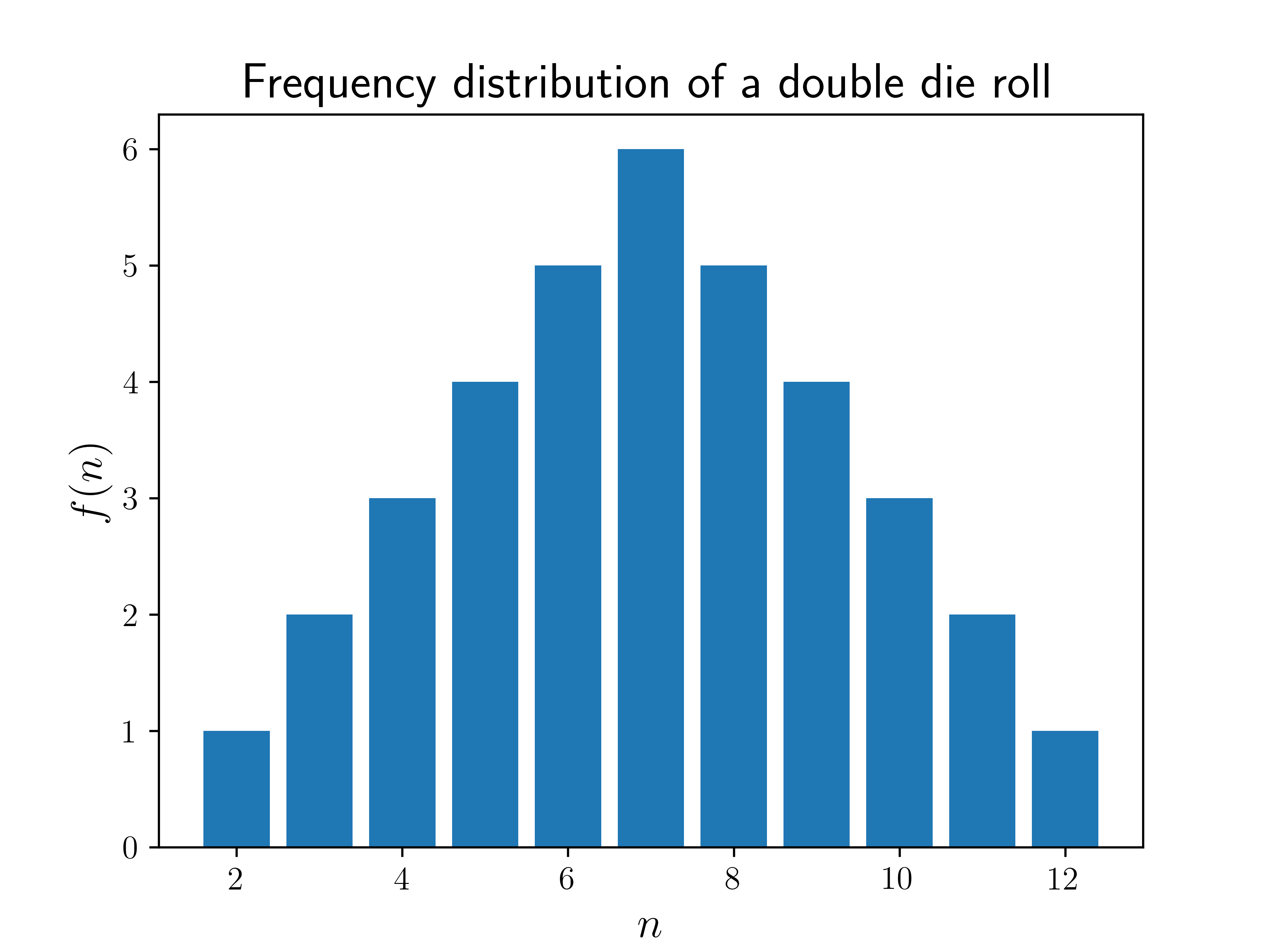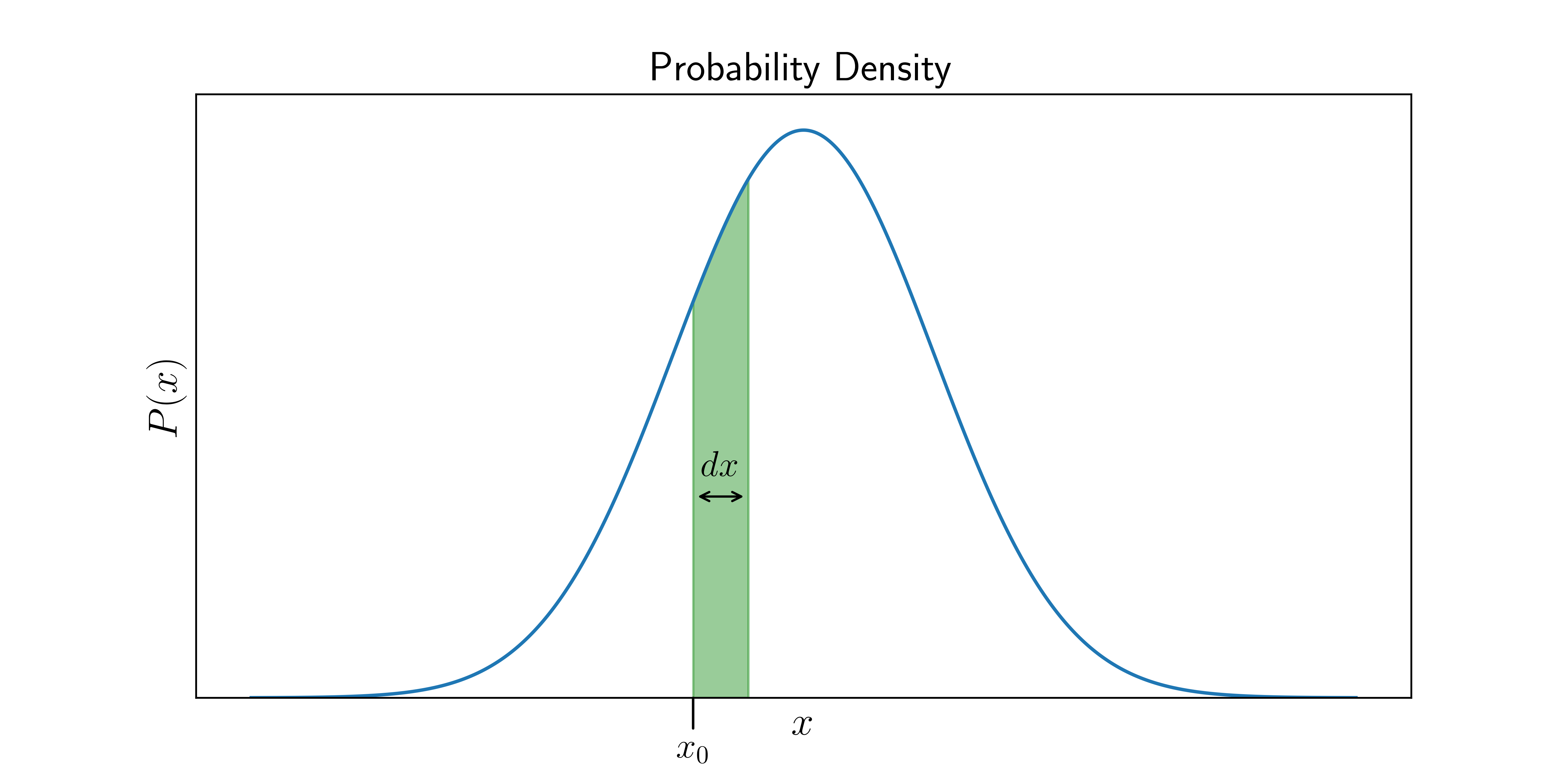What's a probability distribution?

Probability distributions are mathematical functions that give the probabilities of a range or set of outcomes. These outcomes can be the result of an experiment or procedure, such as tossing a coin or rolling dice. They can also be the result of a physical measurement, such as measuring the temperature of an object, counting how many electrons are spin up, etc. Broadly speaking, we can classify probability distributions into two categories - discrete probability distributions and continuous probability distributions.

Discrete Probability Distributions

It's intuitive for us to understand what a discrete probability distribution is. For example, we understand the outcomes of a coin toss very well, and also that of a dice roll. For a single coin toss, we know that the probability of getting heads is half, or . Similarly, the probability of getting tails is . Formally, we can write the probability distribution for such a coin toss as,

Here, denotes the outcome, and we used the "set notation", , which means " belongs to a set containing and ". From the above equation, we can also assume that any other outcome for (such as landing on an edge) is incredibly unlikely, impossible, or simply "not allowed" (for example, just toss again if it does land on its edge!).

For a probability distribution, it's important to take note of the set of possibilities, or the domain of the distribution. Here, is the domain of , telling us that can only be either or .

If we use a different system, the outcome could mean other things. For example, it could be a number like the outcome of a die roll which has the probability distribution,

This is saying that the probability of being a whole number between and is , and we assume that the probability of getting any other is . This is a discrete probability function because is an integer, and thus only takes discrete values.

Both of the above examples are rather boring, because the value of is the same for all . An example of a discrete probability function where the probability actually depends on , is when is the sum of numbers on a roll of two dice. In this case, is different for each as some possibilities like can happen in only one possible way (by getting a on both dice), whereas can happen in ways ( and ; or and ; or and ).

The example of rolling two dice is a great case study for how we can construct a probability distribution, since the probability varies and it is not immediately obvious how it varies. So let's go ahead and construct it!

Let's first define the domain of our target . We know that the lowest sum of two dice is (a on both dice), so for sure. Similarly, the maximum is the sum of two sixes, or , so also.

So now we know the domain of possibilities, i.e., . Next, we take a very common approach - for each outcome , we count up the number of different ways it can occur. Let's call this number the frequency of , . We already mentioned that there is only one way to get , by getting a pair of s. By our definition of the function , this means that . For , we see that there are two possible ways of getting this outcome: the first die shows a and the second a , or the first die shows a and the second a . Thus, . If you continue doing this for all , you may see a pattern (homework for the reader!). Once you have all the , we can visualize it by plotting vs , as shown below.We can see from the plot that the most common outcome for the sum of two dice is a , and the further away from you get, the less likely the outcome. Good to know, for a prospective gambler!

Normalization

The plotted above is technically NOT the probability – because we know that the sum of all probabilities should be , which clearly isn't the case for . But we can get the probability by dividing by the total number of possibilities, . For two dice, that is , but we could also express it as the sum of all frequencies,

which would also equal to in this case. So, by dividing by we get our target probability distribution, . This process is called normalization and is crucial for determining almost any probability distribution. So in general, if we have the function , we can get the probability as

Note that does not necessarily have to be the frequency of – it could be any function which is proportional to , and the above definition of would still hold. It's easy to check that the sum is now equal to , since

Once we have the probability function , we can calculate all sorts of probabilites. For example, let's say we want to find the probability that will be between two integers and , inclusively (also including and ). For brevity, we will use the notation to denote this probability. And to calculate it, we simply have to sum up all the probabilities for each value of in that range, i.e.,

Probability Density Functions

What if instead of a discrete variable , we had a continuous variable , like temperature or weight? In that case, it doesn't make sense to ask what the probability is of being exactly a particular number – there are infinite possible real numbers, after all, so the probability of being exactly any one of them is essentially zero! But it does make sense to ask what the probability is that will be between a certain range of values. For example, one might say that there is chance that the temperature tomorrow at noon will be between and , or chance that it will be between and . But how do we put all that information, for every possible range, in a single function? The answer is to use a probability density function.

What does that mean? Well, suppose is a continous quantity, and we have a probability density function, which looks likeNow, if we are interested in the probability of the range of values that lie between and , all we have to do is calculate the area of the green sliver above. This is the defining feature of a probability density function:

the probability of a range of values is the area of the region under the probability density curve which is within that range.

So if is infinitesimally small, then the area of the green sliver becomes , and hence,

So strictly speaking, itself is NOT a probability, but rather the probability is the quantity , or any area under the curve. That is why we call the probability density at , while the actual probability is only defined for ranges of .

Thus, to obtain the probability of lying within a range, we simply integrate between that range, i.e.,

This is analagous to finding the probability of a range of discrete values from the previous section:

The fact that all probabilities must sum to translates to

where denotes the domain of , i.e., the entire range of possible values of for which is defined.

Normalization of a Density Function

Just like in the discrete case, we often first calculate some density or frequency function , which is NOT , but proportional to it. We can get the probability density function by normalizing it in a similar way, except that we integrate instead of sum:

For example, consider the following Gaussian function (popularly used in normal distributions),

which is defined for all real numbers . We first integrate it (or do a quick google search, as it is rather tricky) to get

Now we have a Gaussian probability distribution,

In general, normalization can allow us to create a probability distribution out of almost any function . There are really only two rules that must satisfy to be a candidate for a probability density distribution:

1. The integral of over any subset of (denoted by ) has to be non-negative (it can be zero):
2. The following integral must be finite:

Text